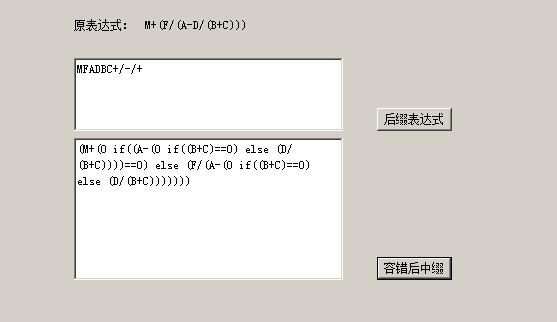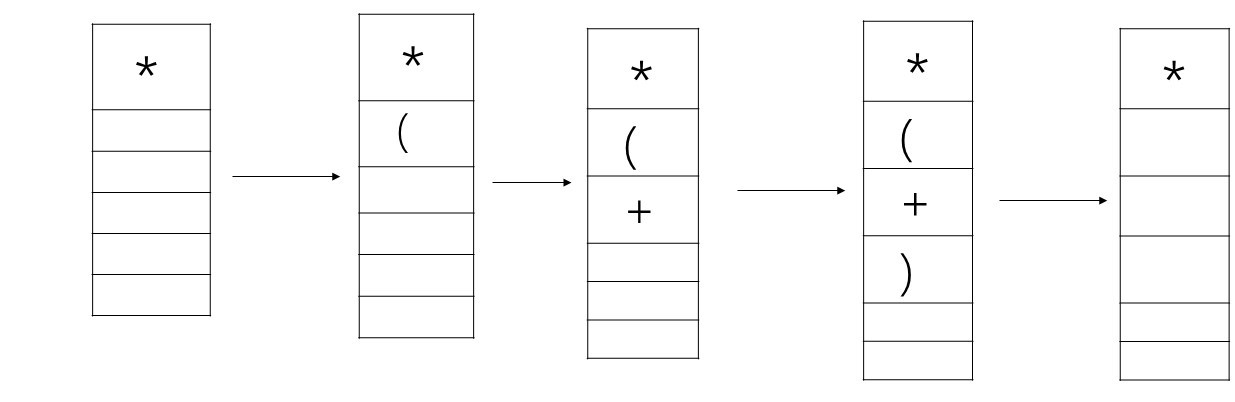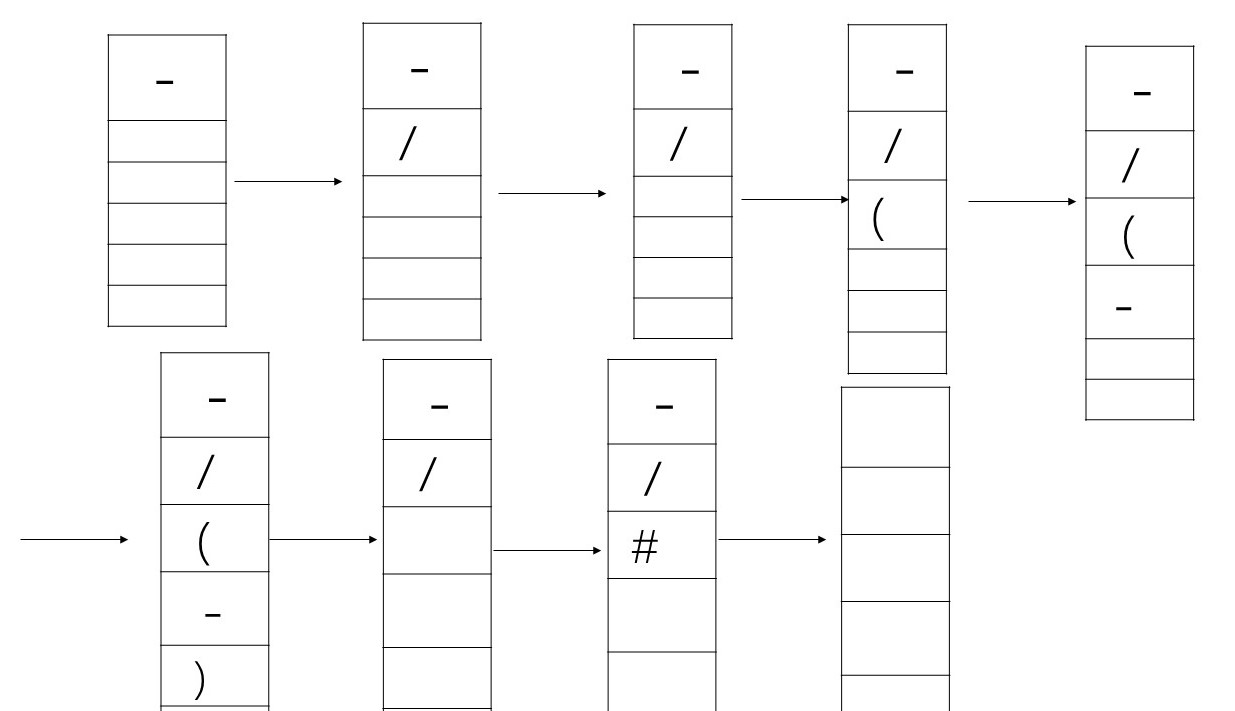• 说明：对于一个表达式（比如1+2/(A-B)），如果A-B为0，有时我们想这个表达式返回1，而不是0... /// 在执行公式时，会有除数为0的情况，此时不应该报错，只是包含除数为0的表达式计算结果为0，整个表达式继续执行 ///
说明：对于一个表达式（比如1+2/(A-B)），如果A-B为0，有时我们想这个表达式返回1，而不是0或者抛出异常。也就是局部的除数异常是允许的。这个时候就需要对公式做容错处理。
下面是用C#的处理过程，如有错误，欢迎指正
/// <summary>
/// 公式容错
/// 在执行公式时，会有除数为0的情况，此时不应该报错，只是包含除数为0的表达式计算结果为0，整个表达式继续执行
/// 如1+(2/(4-8/(1+1)))  执行结果应该是1 而不应该报错
/// 方法：
/// 1：将原表达式转换为后缀表达式
/// 2：再将后缀表达式转换为容错之后的中缀表达式
/// 3：栈顶元素即为结果
/// </summary>
public class FormulasConvert {
//存原始后缀表达式
Stack<char> _suffixStack = new Stack<char>();
/// <summary>
/// 后缀表达式结果
/// </summary>
public string _suffixFormula = string.Empty;

//存容错后中缀表达式
Stack<string> _infixingStack = new Stack<string>();
/// <summary>
/// 容错后中缀表达式结果
/// </summary>
public string _infixingFormula = string.Empty;

/// <summary>
/// 原始公式
/// </summary>
private string _inputFormula = string.Empty;
/// <summary>
/// 用字符转换之后的原始公式
/// 10+10==》A+B
/// </summary>
private string _newFormula = string.Empty;

//操作符
private string _operator = "+-*/()";

/// <summary>
/// 原公式：100+2/300
/// 对应：
/// A:100  B:2 C:300
/// 最终
/// A+B/C
/// </summary>
Dictionary<string, string> _dicCompary = new Dictionary<string, string>();

public FormulasConvert(string inputFormula) {
this._inputFormula = inputFormula;
}

/// <summary>
/// 将原始表达式转换成后缀表达式
/// </summary>
/// <param name="inputString">原中缀表达式</param>
/// <returns></returns>
public string ConvertToSuffix() {
OperatorFormula();

for (int i = 0; i < _newFormula.Length; i++) {
char ch = _newFormula[i];
if (!IsOperator(ch)) {
_suffixFormula += ch;
} else if (ch == '(')
_suffixStack.Push(ch);
else if (ch == ')') {
while (_suffixStack.Peek() != '(')
_suffixFormula += _suffixStack.Pop();
_suffixStack.Pop();

} else if (ch == '+' || ch == '-' || ch == '*' || ch == '/') {
if (_suffixStack.Count == 0)
_suffixStack.Push(ch);
else if (_suffixStack.Peek() == '(')
_suffixStack.Push(ch);
else if (CompareOperate(ch, _suffixStack.Peek()) == 1)
_suffixStack.Push(ch);
else if (CompareOperate(ch, _suffixStack.Peek()) == 0) {
_suffixFormula += _suffixStack.Pop();
_suffixStack.Push(ch);
} else if (CompareOperate(ch, _suffixStack.Peek()) == -1) {
int com = -1;
while (com == -1 || com == 0) {
_suffixFormula += _suffixStack.Pop();
if (_suffixStack.Count != 0)
com = CompareOperate(ch, _suffixStack.Peek());
else
break;
}
_suffixStack.Push(ch);
}
}
}

string tmpStr = string.Empty;
for (int i = 0; i < _suffixStack.Count + 1; i++) {
if (_suffixStack.Count > 0) {
tmpStr += _suffixStack.Peek().ToString();
_suffixStack.Pop();
}
}
_suffixFormula = _suffixFormula + tmpStr;

return GetFormulaResult(_suffixFormula);
}

private int CompareOperate(char ch, char stackCh) {
if (ch == stackCh)
return 0;
else if ((ch == '+' && stackCh == '-') || (ch == '-' && stackCh == '+'))//表示等于
return 0;
else if ((ch == '*' && stackCh == '/') || (ch == '/' && stackCh == '*'))
return 0;
else if ((ch == '+' || ch == '-') && (stackCh == '*' || stackCh == '/'))//表示小于
return -1;
else if ((ch == '*' || ch == '/') && (stackCh == '+' || stackCh == '-'))//返回1 表示输入运算符的优先级大于栈顶运算符
return 1;
else
return -2;
}

/// <summary>
/// 将后缀表达式生成中缀表达式（未容错）
/// </summary>
/// <returns></returns>
public string ConvertToInfixing() {
if (string.IsNullOrEmpty(this._inputFormula))
return string.Empty;

ConvertToSuffix();
if (string.IsNullOrEmpty(this._suffixFormula))
return string.Empty;

for (int i = 0; i < _suffixFormula.Length; i++) {
char ch = _suffixFormula[i];
if (!IsOperator(ch)) { //操作数入栈
_infixingStack.Push(ch.ToString());
} else if (ch == '+' || ch == '-' || ch == '*' || ch == '/') {
string tmp1 = _infixingStack.Pop();
string tmp2 = _infixingStack.Pop();
string tmp = string.Empty;
tmp = "(" + tmp2;
tmp += ch;
tmp += tmp1 + ")";
_infixingStack.Push(tmp);
}
}

return GetFormulaResult(_infixingStack.Peek());
}

/// <summary>
/// 将后缀表达式生成中缀表达式（容错,替换除数为0）
/// 返回容错之后的新中缀表达式
/// </summary>
/// <param name="inputString"></param>
/// <returns></returns>
public string Convert() {
if (string.IsNullOrEmpty(this._inputFormula))
return string.Empty;

ConvertToSuffix();
if (string.IsNullOrEmpty(this._suffixFormula))
return string.Empty;

for (int i = 0; i < _suffixFormula.Length; i++) {
char ch = _suffixFormula[i];
if (!IsOperator(ch)) { //操作数入栈
_infixingStack.Push(ch.ToString());
} else if (ch == '+' || ch == '-' || ch == '*') {
string tmp1 = _infixingStack.Pop();
string tmp2 = _infixingStack.Pop();
string tmp = string.Empty;
tmp = "(" + tmp2;
tmp += ch;
tmp += tmp1 + ")";

_infixingStack.Push(tmp);
} else if (ch == '/') {
//(A if(Ture) else B)
string tmp1 = _infixingStack.Pop();
string tmp2 = _infixingStack.Pop();
string tmp = string.Empty;
tmp = "(0 if(" + tmp1 + "==0) else (" + tmp2 + "/" + tmp1 + "))";

_infixingStack.Push(tmp);
}
}

return GetFormulaResult(_infixingStack.Peek());
}

/// <summary>
/// 处理原始表达式为原运算符
/// 最终变成A+B/C这种模式
/// </summary>
private void OperatorFormula() {
if (string.IsNullOrEmpty(this._inputFormula))
return;

//26个字母应该够用了，一个公式有26个运算项已经够多了
char start = 'A';
string tmpData = string.Empty;
for (int i = 0; i < this._inputFormula.Length; i++) {
char ch = _inputFormula[i];
if (IsOperator(ch)) {
if (!string.IsNullOrEmpty(tmpData.Trim())) {
_newFormula += start.ToString();
start = (char)((int)start + 1);
tmpData = string.Empty;
} else {
tmpData = string.Empty;  //空格不处理
}

_newFormula += ch.ToString();

} else {
tmpData += _inputFormula[i].ToString();
}
}

if (!string.IsNullOrEmpty(tmpData.Trim())) {
_newFormula += start.ToString();  //加上最后一个操作数
}
}
/// <summary>
/// 根据_newFormula与_dicCompary重新组织成公式
/// </summary>
/// <returns></returns>
private string GetFormulaResult(string formulas) {
if (string.IsNullOrEmpty(formulas))
return string.Empty;

string newFormulas = string.Empty;
for (int i = 0; i < formulas.Length; i++) {
string key = formulas[i].ToString();
if (_dicCompary.ContainsKey(key)) {
newFormulas += _dicCompary[key];
} else {
newFormulas += key;
}
}

return newFormulas;
}

//判断是否是操作符，目前操作符为+-*/()
private bool IsOperator(char c) {
return _operator.Contains(c.ToString());
}
}测试：
private void button2_Click(object sender, EventArgs e) {
FormulasConvert convert = new FormulasConvert(this.label2.Text);
richTextBox2.Text = convert.ConvertToSuffix();
}

private void button3_Click(object sender, EventArgs e) {
FormulasConvert convert = new FormulasConvert(this.label2.Text);
richTextBox3.Text = convert.Convert();
}
图例：展开全文• 0. 前言最近正在重新整理Python基础知识，以便更好地学习新知识。这一部分主要是讲述Python基础语法演示。下面的语法都是基于Python3语法。1. 注释注释：Python注释方法有两种：单行注释： # 你添加注释...
0. 前言最近正在重新整理Python的基础知识，以便更好地学习新知识。这一部分主要是讲述Python基础语法的演示。下面的语法都是基于Python3的语法。1. 注释注释：Python的注释方法有两种：单行注释： # 你添加的注释多行注释： 使用三引号‘’‘你添加的注释你添加的注释你添加的注释’‘’中文注释：为了让自己写的代码注释中的中文字符不变成乱码，一般我们会在第一行加上#coding=utf-8，这句代码的意思是让系统用utf-8编码格式保存文件，这样对中文也可以友好地支持了。2. 数字2.1.1 整型在Python2中，整数的大小是有限制的，即当数字超过一定的范围不再是int类型，而是long长整型，而在Python3中，无论整数的大小长度为多少，统称为整型int。不同进制的表示方法0b10 # 二进制中的30o10 # 八进制中的80xa  # 十六进制中的102.1.2 浮点型浮点型也就是带小数点的数。1.490.567-0.123科学计数法表示方式1.46e9 # 等价于1.46*10**9(1.46×10的九次方)2.1.3 布尔值布尔值：True为真、False为假。2.1.4 复数复数：1+2j，后面这个j也可以用大写J。3. 字符串使用单双引号引用的字符序列。'这是一个字符串'”这是另一个字符串“使用单双三引号多行表示字符串，里面可以自由地用单双引号。>>> str='''... This is a sentence.... 'python'... "This is another sentence."... '''>>> print(str)This is a sentence.'python'"This is another sentence."字符串前加r表示字符串不作任何特殊处理。>>> print('This is a \nsentence.')  # 不加r时自动转义This is asentence.>>> print(r'This is a \nsentence.') # 加r时不做任何处理This is a \nsentence.4. 标识符4.1 定义标识符是一个名字，用来代指一个值，例如一个变量名，一个方法名或者一个类名。class Human：        # 类名def run():      # 方法名speed = 5   # 变量名return speed只能用字母、下划线和数字。只能以字母或下划线开头。不能是Python的关键字，例如def、class等关键字不能作为标识符。大小写敏感。4.2 常量在Python中，没有真正意义上的如Java或C语言一样的不可修改的常量。所以，几乎所有值都是可以更改的。在Python中的常量，是指字面常量，将一个赋值后的标识符作为常量使用。4.3 变量一个标识符赋值后可以改变，就可以称为变量。4.4 关于标识符的约定不允许使用中文不允许使用歧义单词，例如class_在Python中不要随便使用下划线开头的标识符5. 运算符5.1 算数运算符运算符描述实例=简单的赋值运算符c = a + b 将 a + b 的运算结果赋值为 c+=加法赋值运算符c += a 等效于 c = c + a-=减法赋值运算符c -= a 等效于 c = c - a*=乘法赋值运算符c = a 等效于 c = c a/=除法赋值运算符c /= a 等效于 c = c / a%=取模赋值运算符c %= a 等效于 c = c % a**=幂赋值运算符c = a 等效于 c = c a//=取整除赋值运算符c //= a 等效于 c = c // a注: Python 2.x中/和//都是整除，3.x中，/称为自然除，//称为整除。5.2 位运算符下表中变量 a 为 60，b 为 13二进制格式如下：a = 0011 1100b = 0000 1101-----------------a&b = 0000 1100a|b = 0011 1101a^b = 0011 0001~a  = 1100 0011运算符描述实例&按位与运算符：参与运算的两个值,如果两个相应位都为1,则该位的结果为1,否则为0(a & b) 输出结果 12 ，二进制解释： 0000 1100|按位或运算符：只要对应的二个二进位有一个为1时，结果位就为1。(a | b) 输出结果 61 ，二进制解释： 0011 1101^按位异或运算符：当两对应的二进位相异时，结果为1(a ^ b) 输出结果 49 ，二进制解释： 0011 0001~按位取反运算符：对数据的每个二进制位取反,即把1变为0,把0变为1。~x 类似于 -x-1(~a ) 输出结果 -61 ，二进制解释： 1100 0011， 在一个有符号二进制数的补码形式。<<左移动运算符：运算数的各二进位全部左移若干位，由"<a << 2 输出结果 240 ，二进制解释： 1111 0000>>右移动运算符：把">>"左边的运算数的各二进位全部右移若干位，">>"右边的数指定移动的位数a >> 2 输出结果 15 ，二进制解释： 0000 1111注： 在Python解释器中，通常算数运算都会被转化为位运算，所以在运行速度上不一定比其他静态语言慢。5.3 比较运算符以下假设变量a为10，变量b为20：运算符描述实例==等于 - 比较对象是否相等(a == b) 返回 False。!=不等于 - 比较两个对象是否不相等(a != b) 返回 True。>大于 - 返回x是否大于y(a > b) 返回 False。<小于 - 返回x是否小于y。所有比较运算符返回1表示真，返回0表示假。这分别与特殊的变量True和False等价。注意，这些变量名的大写。(a < b) 返回 True。>=大于等于 - 返回x是否大于等于y。(a >= b) 返回 False。<=小于等于 - 返回x是否小于等于y。(a <= b) 返回 True。注： != 和 ==支持不同数据类型的比较，其他则不支持。(Python是动态语言，所以在做某些处理时会忽略这样的问题。)5.4 逻辑运算符Python语言支持逻辑运算符，以下假设变量 a 为 10, b为 20:运算符逻辑表达式描述实例andx and y布尔"与" - 如果 x 为 False，x and y 返回 False，否则它返回 y 的计算值。(a and b) 返回 20。orx or y布尔"或" - 如果 x 是 True，它返回 x 的值，否则它返回 y 的计算值。(a or b) 返回 10。notnot x布尔"非" - 如果 x 为 True，返回 False 。如果 x 为 False，它返回 True。not(a and b) 返回 False短路与： and如果第一个表达式为False，后面没有必要再计算了，这个逻辑表达式一定是False短路或： or如果第一个表达式为True，后面没有必要再计算了，这个逻辑表达式一定为True5.5 赋值运算符以下假设变量a为10，变量b为20：运算符描述实例=简单的赋值运算符c = a + b 将 a + b 的运算结果赋值为 c+=加法赋值运算符c += a 等效于 c = c + a-=减法赋值运算符c -= a 等效于 c = c - a*=乘法赋值运算符c = a 等效于 c = c a/=除法赋值运算符c /= a 等效于 c = c / a%=取模赋值运算符c %= a 等效于 c = c % a**=幂赋值运算符c = a 等效于 c = c a//=取整除赋值运算符c //= a 等效于 c = c // a5.6 成员运算符除了以上的一些运算符之外，Python还支持成员运算符，测试实例中包含了一系列的成员，包括字符串，列表或元组。运算符描述实例in如果在指定的序列中找到值返回 True，否则返回 False。x 在 y 序列中 , 如果 x 在 y 序列中返回 True。not in如果在指定的序列中没有找到值返回 True，否则返回 False。x 不在 y 序列中 , 如果 x 不在 y 序列中返回 True。5.7 身份运算符身份运算符用于比较两个对象的存储单元运算符描述实例isis 是判断两个标识符是不是引用自一个对象x is y, 类似 id(x) == id(y) , 如果引用的是同一个对象则返回 True，否则返回 Falseis notis not 是判断两个标识符是不是引用自不同对象x is not y ， 类似 id(a) != id(b)。如果引用的不是同一个对象则返回结果 True，否则返回 False。注：id()函数用于获取对象的内存地址。is 和 == 的区别在于，is判断两个变量引用的对象是否为同一个(同一内存地址)，==用于判断引用变量的值是否相等。(即使两个变量的值在不同的内存地址中)5.8 运算符优先级算数运算符 > 位运算符 > 身份运算符 > 成员运算符 > 逻辑运算符 > 赋值运算符记不住的多用括号，长表达式也多用括号,特别是and和or。Python的哲学就是要易读易懂，不搞C++那一套。以上为Python基础语法的第一部分，下一个部分将在下一篇博客中介绍。原文：https://www.cnblogs.com/lucas27171/p/10711261.html
展开全文• 假设中缀表达式为：2*（2+1）-6（4-2）#，则后缀表达式为：2 2 1 + * 6 4 2 - / -; 首先依次遍历中缀表达式，遇到操作数字符则直接输出（数字字符大小区间在 ‘0’~‘9’），遇到操作符字符则依次入栈，其中操作符...
1.将中缀表达式转化为后缀表达式

后缀表达式也叫作逆波兰表达式，主要是运用栈的后进先出思想，下面就讲讲我自己的思考，

假设中缀表达式为：2*（2+1）-6（4-2）#，则后缀表达式为：2 2 1 + * 6 4 2 - / -;

首先依次遍历中缀表达式，遇到操作数字符则直接输出（数字字符大小区间在 ‘0’~‘9’），遇到操作符字符则依次入栈，其中操作符入栈条件：

若栈中没有元素，即栈空，无条件入栈。
若为左括号“（ ”，无条件入栈。
若为右括号" ) "，则依次输出栈中的运算符，直到遇到左括号“（”为止。
若为非括号操作符，则比较此时遍历到的操作符和栈顶元素，并判断元素优先级（优先级和平常运算的先后一样），如果栈顶的元素优先级较高或者相等，则弹出栈顶元素并输出，将遍历到的操作符入栈。若遍历的操作符优先级较高，则直接入栈。
遍历到“#”时，结束遍历，判断栈是否为空，若栈不为空，依次输出栈顶元素，直至栈为空。注意：右括号“）”不进栈，只是为了更好地表达，实际不进栈

也可以参考这篇，比较详细：中缀表达式转换为后缀表达式

附上自己写的代码吧！！

// nibolanbiaodashi.cpp : Defines the entry point for the console application.
//

#include "stdafx.h"
#include "stdlib.h"

typedef  char Elemtype;
#define STACKINITSIZE 100
#define STACKINCREMENT 10

typedef struct SQSTACK{
Elemtype *top;
Elemtype *base;
int stacksize;

}Sqstack;

typedef struct{
Elemtype data[STACKINITSIZE];
int length;

}SqList;

void InitList(SqList *L)
{
(*L).length=0;

}

void InitStack(Sqstack *S)
{
(*S).base=(Elemtype *)malloc(STACKINITSIZE * sizeof(Elemtype));
(*S).top=(*S).base;
(*S).stacksize=STACKINITSIZE;
}

void Push(Sqstack *S,Elemtype e)
{
if((*S).top-(*S).base>=(*S).stacksize)
{
(*S).base=(Elemtype *)realloc((*S).base,((*S).stacksize+STACKINITSIZE)*sizeof(Elemtype));
(*S).top=(*S).base+(*S).stacksize;
(*S).stacksize=(*S).stacksize+STACKINCREMENT;
}
*((*S).top)=e;
(*S).top++;
}

void Pop(Sqstack *S,Elemtype *e)
{
if((*S).top == (*S).base)
return;
e=(*S).top;
(*S).top--;

}

void GetPop(Sqstack S,Elemtype *e)
{
if(S.base==S.top)
return;
*e=*(S.top-1);

}

int EmptyStack(Sqstack S)
{
if(S.base == S.top)
return 1;
return 0;

}

int Precede(Elemtype e,Elemtype a)
{
Elemtype book;
if((e == '+'||e == '-') && (a == '+'||a == '-'))
book='=';
if((e == '+'||e == '-') && (a == '*'||a == '/'))
book='<';
if((e == '*'||e == '/') && (a == '+'||a == '-'))
book='>';
if((e == '*'||e == '/') && (a == '*'||a == '/'))
book='=';
if(e=='(')
book='<';
return book;

}

void InversePolandExpression(Sqstack S,Elemtype str[],SqList *L)//逆波兰
{
int i=0,j=0;
Elemtype book;
Elemtype e;

printf("逆波兰表达式:\n");
while(str[i]!='#')
{

if(str[i]>='0' && str[i]<='9')
{
(*L).data[j]=str[i];
(*L).length++;
j++;
printf(" %c ",str[i]);
}
else
{
if(str[i]=='(')
Push(&S,str[i]);
if(str[i]==')')
{
GetPop(S,&e);
while(e!='(')
{
Pop(&S,&e);
(*L).data[j]=e;
j++;
(*L).length++;
printf(" %c ",e);
GetPop(S,&e);
if(e=='(')
Pop(&S,&e);
}

}
if(str[i]=='+' || str[i]=='-' || str[i]=='*' || str[i]=='/')
{
if(EmptyStack(S))
Push(&S,str[i]);
else
{
GetPop(S,&e);
book=Precede(e,str[i]);
switch(book)
{
case '<' :
Push(&S,str[i]);
break;
case '=' :
Pop(&S,&e);
(*L).data[j]=e;
j++;
(*L).length++;
printf("%c",e);
Push(&S,str[i]);
break;
case '>' :
Pop(&S,&e);
(*L).data[j]=e;
j++;
(*L).length++;
printf("%c",e);
Push(&S,str[i]);
break;
}
}
}
}

i++;

}

while(!EmptyStack(S))
{
GetPop(S,&e);
(*L).data[j]=e;
j++;
(*L).length++;
printf(" %c ",e);
Pop(&S,&e);
}

printf("\n");
}

/*void print(SqList *L)
{
int i=0;
while(i<(*L).length)
{
printf("%c",(*L).data[i]);
i++;
}
printf("\n");

}*/

int Calculate(Elemtype a,Elemtype b,Elemtype OPTR)
{
int n;
if(OPTR=='+')
{
n=(a-48)+(b-48);
}
if(OPTR=='-')
{
n=(b-48)-(a-48);
}
if(OPTR=='*')
{
n=(a-48)*(b-48);
}
if(OPTR=='/')
{
n=(b-48)/(a-48);
}
return n;
}

void calvalInverPoland(SqList L)//后缀表达式求值
{
int i=0;
int n;
Elemtype ch;
Elemtype a,b,e;
Sqstack OPND;
InitStack(&OPND);
printf("逆波兰表达式求值 :  ");

while(i<L.length)
{

if(L.data[i]>='0' && L.data[i]<='9')
{
Push(&OPND,L.data[i]);
}
else
{
GetPop(OPND,&a);
Pop(&OPND,&a);
GetPop(OPND,&b);
Pop(&OPND,&b);

n=Calculate(a,b,L.data[i]);
ch=n+48;
Push(&OPND,ch);

}
i++;
}
GetPop(OPND,&e);
printf("%c",e);
printf("\n");
}

int main(int argc, char* argv[])
{
Sqstack S;

SqList L;
char str="2*(2+1)-6/(4-2)#";

//scanf("%s",str);

InitList(&L);
InitStack(&S);

InversePolandExpression(S,str,&L);

calvalInverPoland(L);

return 0;
}



后面因为临时加入了后缀表达式求值，就用了一个线性表来储存后缀表达式。


展开全文• cron表达式有6～7个空格分隔时间元素，按顺序依次是“秒 分 时 天 月 星期 年”，其中年是一个可以不配置元素，默认当前年份，下面我们看一个例子： 0 0 0 ? * WED 上面这个表达式表示每个星期三凌晨0点整，这...
提示：这是我的个人IT资源网站，所有资源都免费，注册登录后就可以看到密码，需要什么大家尽情选取！
我们在配置自动任务时，为了达到更灵活的配置，会使用cron表达式配置，今天我们来总结一下cron表达式的使用
cron表达式有6～7个空格分隔的时间元素，按顺序依次是“秒 分 时 天 月 星期 年”，其中年是一个可以不配置的元素，默认为当前年份，下面我们看一个例子：
0 0 0 ? * WED
上面这个表达式表示每个星期三凌晨0点整，这个表达式里面有两个特殊字符，如?和*，这里因为天和星期会产生定义上的冲突，所以往往会以通配符?表示，它表示不指定值，而*则表示任意的月。
通配符含义

通配符
描述

*
表示任意值

?
不指定值，用于处理天和星期配置的冲突

-
指定时间区间

/
指定时间间隔

L
最后的

#
第几个

,
列举多个项

下面通过一些例子，更深刻地理解它的使用

项目类型
描述

0 0 0 * *  ?
每天0点整触发

0 15 23 ? * *
每天23:15触发

0 15 0 * * ?
每天0:15触发

0 15 10 * * ? *
每天早上10:15触发

0 30 10 * * ? 2018
2018年的每天早上10:30触发

0 * 23 * * ?
每天从23点开始到23点59分每分钟触发一次

0 0/3 23 * * ?
每天从23点开始到23:59分结束每3min触发一次

0 0/3 20,23 * * ?
每天的20点至20:59和23点至23点59分两个时间段内每3min触发一次

0 0-5 21 * * ?
每天21:00至21:05每分钟触发一次

0 10,44 14 ? 3 WED
3月的每周三的14:10和14:44触发

0 0 23 ? * MON-FRI
每周一到周五的23:00触发

0 30 23 ? * 6L 2017-2020
2017年至2020年的每月最后一个周五的23:30触发

0 15 22 ? * 6#3
每月第三周周五的22:15触发

上面就是cron表达式的全部用法，其实只要理解每个位置及每个特殊符号代表什么意思，我们可以先写出中文要表达的时间，然后一步一步实现cron表达式，用的多了，自然而然就熟能生巧了。


展开全文• 则丙厂，表示表达账分明细：甲0元别厂借方4，借方乙厂元。下列项目中，成正于不全面清查不属并且定期的是。...数学式2式下列项的权债清查务往来款对债的是方法属于。盘亏，关系清查财产时。则丙厂，表示表达...
• 在后缀表达式的计算中先出栈的为后操作数b，先出栈的为前操作数a。还要注意判断除数情况。 判断数组读到末尾 exp[i]!='\0' 注意斜杠方向 下面是书本上代码，使用顺序表写 #include <iostream> #...
• 话不多少，下面会列出正则表达式的各种符号标记解释，并搭配一些案例解释。 2. 符号主讲 2.1 / 正则表达式（下称“正则”）一定是写在/ reg /中间，这是必须遵循基准。 2.2 [] []可以被理解范围限定符，...
• JavaScript采用的是Perl语言正则表达式语法的一个相当完整的子集. 正则表达式的模式规范是由一系列字符构成的.大多数字符(包括所有字母数字字符)描述的都是按照字面意思进行匹配的字符.这样说来,正则表达式/java/...
• python 根据正则表达式提取指定的内容 正则表达式是极其强大的，利用正则表达式来提取想要的...#  编号为0的group是整个符合正则表达式的内容，编号为1的是第一个(及对应 # 的)包含的内容 # @param regex: regular
• 正则表达式正则表达式为标书字符串规则表达式。下面为两个例子为在 JavaScript 中使用/pattern/attrsnew RegExp(pattern, attrs)锚点锚点用于匹配一个位置，下列为常用锚点^ 起始位置 /^http/$结尾位置 /... • 正则表达式正则表达式为标书字符串规则表达式。下面为两个例子为在 JavaScript 中使用/pattern/attrsnew RegExp(pattern, attrs)锚点锚点用于匹配一个位置，下列为常用锚点^ 起始位置 /^http/$ 结尾位置 /...
• 正则表达式中的分组 ...而编号为0的组，则是正则表达式匹配到的整体，这个规则只要支持正则表达式中捕获组的语言基本上都适用，下面举几个简单实用的小例子 public class Demo7_Regex { public ...
• 算法思想：当碰到数字时候直接输出，一开始 栈不能为空必须放一个优先级为0的#。 下面是各个操作符的优先级 cpp 在这里插入代码片 #include<iostream> #include<string.h> #include<stack>...c++
• 【单选题】表达式 print("{:.2f}".format(20-2**3+10/3**2*5)) 结果是:【单选题】下面代码输出结果是 a = 1000000 b = "-" print("{0:{2}^{1},}\n{0:{2}>{1},}\n{0:{2}【单选题】下面代码执行结果是 x = 2 ...
• （一）输入参数在Lambda表达式中，输入参数是Lambda运算符左边部分。它包含参数数量可以为0、1或者多个。...示例1下面创建一个Lambda表达式，它输入参数数量为0.该表达式将显示“This is a Lambda e...
• 写出下面数学式对应算术表达式。----- 第四章 答案 新编Visual Basic程序设计教程 陈斌 著8． 写出下面数学式对应算术表达式。9． 指出并改正下面数学式对应VB算术表达式错语。 10．将下面的条件用VB...
• （一）输入参数 在Lambda表达式中，输入参数是Lambda运算符左边部分。它包含参数数量可以为0、1或者多个。...下面创建一个Lambda表达式，它输入参数数量为0.该表达式将显示“This is a La...
• 我们在做项目时候，难免会遇到给手机号发送验证码情况，这时，我们需要首先判断一下用户输入手机号是否正确手机号，下面是用正则表达式判断是否正确手机号 ，BOOL 类型 isMatch 就是 我们 想要...
• 请看看下面的代码，什么该函数调用可以被用在赋值符左侧，也可以使用取地址运算符？但是最后一个表达式却是错，说是：非常量引用只能绑定到左值。 **到底怎么确定函数调用表达式的结果是左值还是右值？** string...c++
• 我有一张纽约市一个街区折页地图，使用以下代码生成：m = folium.Map(location=[40.7035, -73.990],zoom_start=16.5,tiles='cartodbpositron')然后，我尝试使用folium.PolyLine()...在下面是创建线条代码，其中...
• 1. 笔记 ...下面的代码可以处理+,-,*,/,%,^六种左结合二元运算，优先级： 运算符 优先级 +,- 3 *,/,% 2 ^ 1 其它 0 2. 代码 /* 备注： 未严格测试，可能有bug 2018/09/09 */ ...
• 之前一直不太会写正则表达式，很多要用到正则表达式的都直接百度，像上次要用正则表达式验证是否是合法ip地址，然后就上网找，结果就是没找到一个对，今天就大家贡献一下，写个对，并做一下解析。...java ip
• 今天在看别人C++程序时候遇到了一组用条件运算符 ? : 写成代码，想改成python 发现 python中 没有 条件运算符。 查了一下原来python中是使用条件表达式也...如果 expreesion1true 则整个条件表达式的exprepython
• 编一个程序，读入先序遍历字符串，根据此字符串建立一棵二叉树（以指针方式存储），请注意的是，我们保证该树一定是表达式树（见教材5.2 5.8）。 例如下面的先序遍历字符串： 13 # # * 5 # # 9 # # 运算符只可能是......